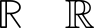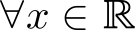# 使用 unicode-math 宏包时 \mathbb 黑板体字符的显示问题## 解决方案

\documentclass{article}
\usepackage{amssymb}
\let\mathbbalt\mathbb
\usepackage{unicode-math}
% \let\mathbb\mathbbalt % UNIVERSAL RESET TO ORIGINAL \mathbb
\begin{document}
\begin{equation}
\mathbb{R}\quad\mathbbalt{R} % OR JUST CALL ON INDIVIDUAL ORIGINAL GLYPHS
\end{equation}
\end{document}


\documentclass{article}
\usepackage{unicode-math}
\setmathfont{LatinModernMath-Regular}
\setmathfont[range=\mathbb]{TeXGyrePagellaMath-Regular}
\begin{document}
$\forall x \in \mathbb{R}$
\end{document}